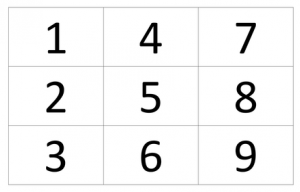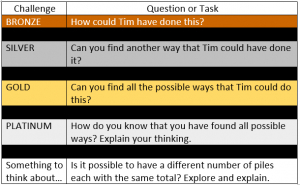# Maths Challenge – Can you make fifteen?

Tim had nine cards, each with a different number from 1 to 9 on it.He put the cards into three piles so that the total in each pile was 15.Hints and Tips

2. Think about the total of the whole set of cards.
3. You don’t have to have the same amount of cards in each pile. Only the total of each pile should be the same.

### 10 thoughts on “Maths Challenge – Can you make fifteen?”

•23rd March 2020 at 11:28 am

Bronze: 7+8=15 6+5+4=15 1+2+3+9=15

Silver: 7+8=15 6+5+3+1=15 9+2+4=15

Gold: 7+8=15 2+4+5+3+1=15 9+6=15

All you have to do is exchange 1 number for 2 numbers with the same quantity

•23rd March 2020 at 11:45 am

bronze 5+4+6
9+4+2
5+3+7
9+1+5
2+6+7
2+8+5

•23rd March 2020 at 2:04 pm

Hi George, well done for your effort. What will the 3 pile of cards be if each card is used once?

•24th March 2020 at 8:57 am

Well done George. Could you challenge yourself with silver and see if you could find another way?

•23rd March 2020 at 11:49 am

silver
you could multiply them 3×5=15
2×5+5=15

•23rd March 2020 at 12:04 pm

6+9=15
4+5+6
8+7=15

1+5+9=15
8+7+1_1=15
1+6+8=15
2+5+8=15

•23rd March 2020 at 2:03 pm

Well done for finding lots of ways to make 15 using the cards. What could the 3 piles be? Don’t forget that each card will be used once.

•23rd March 2020 at 1:59 pm

Great effort Simar. You have found 3 ways to do this. Are there definitely no more?

•26th March 2020 at 6:06 pm

1+2+3+9=15
7+8=15
5+4+6=15
6+9=15
9+4+2=15
1+5+3+6=15

•